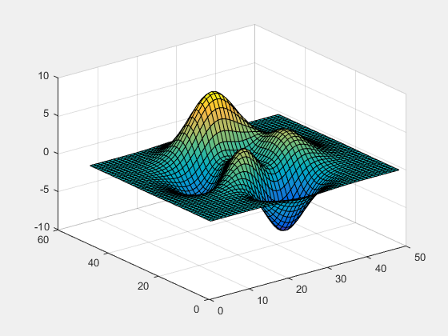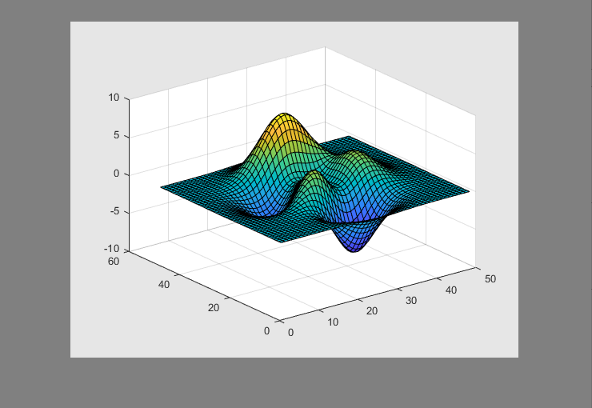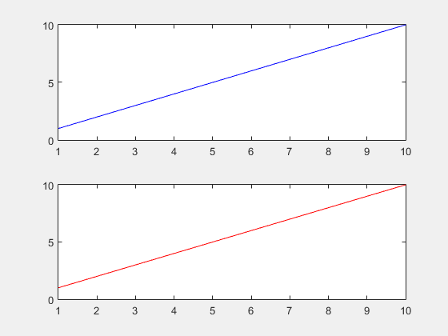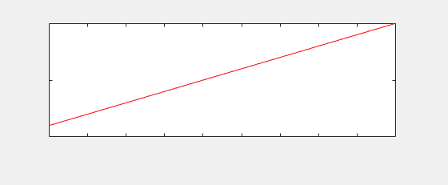Main Content

# getframe

Capture axes or figure as movie frame

## Syntax

``F = getframe``
``F = getframe(ax)``
``F = getframe(fig)``
``F = getframe(___,rect)``

## Description

example

````F = getframe` captures the current axes as it appears on the screen as a movie frame. `F` is a structure containing the image data. `getframe` captures the axes at the same size that it appears on the screen. It does not capture tick labels or other content outside the axes outline.```

example

````F = getframe(ax)` captures the axes identified by `ax` instead of the current axes.```

example

````F = getframe(fig)` captures the figure identified by `fig`. Specify a figure if you want to capture the entire interior of the figure window, including the axes title, labels, and tick marks. The captured movie frame does not include the figure menu and tool bars.```
````F = getframe(___,rect)` captures the area within the rectangle defined by `rect`. Specify `rect` as a four-element vector of the form ```[left bottom width height]```. Use this option with either the `ax` or `fig` input arguments in the previous syntaxes. ```

## Examples

collapse all

Create a plot of random data. Capture the axes and return the image data. `getframe` captures the interior of the axes and the axes outline. It does not capture content that extends beyond the axes outline.

```plot(rand(5)) F = getframe;````F` is a structure with the field `cdata` that contains the captured image data.

Display the captured image data using `imshow`.

```figure imshow(F.cdata)```Create a surface plot. Capture the interior of the figure window, excluding the menu and tool bars.

```surf(peaks) F = getframe(gcf);````F` is a structure with the field `cdata` that contains the captured image data.

Display the captured image data in a figure with a darker background using `imshow`, so you can see captured area.

```figure('Color',[0.5 0.5 0.5]) imshow(F.cdata)```Capture the interior of an axes plus a margin of 30 pixels in each direction. The added margin is necessary to include the tick labels in the capture frame. Depending on the size of the tick labels, the margin might need to be adjusted.

Create a plot of random data.

`plot(rand(5))`Change the axes units to pixels and return the current axes position. The third and fourth elements of the position vector specify the axes width and height in pixels.

```drawnow ax = gca; ax.Units = 'pixels'; pos = ax.Position```
```pos = 73.8000 47.2000 434.0000 342.3000```

Create a four-element vector, `rect`, that defines a rectangular area covering the axes plus the desired margin. The first two elements of `rect` specify the lower left corner of the rectangle relative to the lower left corner of the axes. The last two elements of `rect` specify the width and height of the rectangle. Reset the axes units to the default value of `'normalized'`.

```marg = 30; rect = [-marg, -marg, pos(3)+2*marg, pos(4)+2*marg]; F = getframe(gca,rect); ax.Units = 'normalized';```

Display the captured image data in a figure with a darker background using `imshow`, so you can see captured area.

```figure('Color',[0.5 0.5 0.5]) imshow(F.cdata)```Calculate a margin around the axes so that the captured image data includes the title, axis labels, and tick labels.

Create a plot with a title and an x-axis label.

```plot(rand(5)) xlabel('x values') title('Plot of Random Data')```Change the axes units to pixels and store the `Position` and `TightInset` property values for the axes. The `TighInset` property is a four-element vector of the form `[left bottom right top]`. The values are the margins used around the axes for the tick values and text labels.

```drawnow ax = gca; ax.Units = 'pixels'; pos = ax.Position; ti = ax.TightInset;```

Create a four-element vector, `rect`, that defines a rectangular area covering the axes plus the automatically calculated margin. The first two elements of `rect` specify the lower left corner of the rectangle relative to the lower left corner of the axes. The last two elements of `rect` specify the width and height of the rectangle.

```rect = [-ti(1), -ti(2), pos(3)+ti(1)+ti(3), pos(4)+ti(2)+ti(4)]; F = getframe(ax,rect);```

Display the captured image data in a figure with a darker background using `imshow`, so you can see captured area.

```figure('Color',[0.5 0.5 0.5]) imshow(F.cdata)```Starting in R2019b, you can display a tiling of plots using the `tiledlayout` and `nexttile` functions. Call the `tiledlayout` function to create a 2-by-1 tiled chart layout. Call the `nexttile` function to create the axes objects `ax1` and `ax2`. Plot a line in each axes.

```tiledlayout(2,1) ax1 = nexttile; plot(1:10,'b') ax2 = nexttile; plot(1:10,'r')```Capture the contents of the lower axes. `getframe` captures the interior and border of the plot. It does not capture tick values or labels that extend beyond the outline of the plot.

`F = getframe(ax2);`

Display the captured image data using `imshow`.

```figure imshow(F.cdata)```Record frames of the `peaks` function vibrating by using  `getframe`  in a loop. Preallocate an array to store the movie frames.

```Z = peaks; surf(Z) axis tight manual ax = gca; ax.NextPlot = 'replaceChildren'; loops = 40; F(loops) = struct('cdata',[],'colormap',[]); for j = 1:loops X = sin(j*pi/10)*Z; surf(X,Z) drawnow F(j) = getframe(gcf); end ```

Playback the movie two times.

```fig = figure; movie(fig,F,2)```

## Input Arguments

collapse all

Axes to capture, specified as an `Axes` object or a `GeographicAxes` object. Use this option if you want to capture an axes that is not the current axes.

`getframe` captures the content within the smallest rectangle that encloses the axes outline. If you want to capture all the tick values and labels, then use the `fig` input argument instead.

Example: `F = getframe(ax);`

Figure to capture, specified as a `Figure` object.

Rectangular area to capture, specified as a four-element vector of the form `[left bottom width height]` in pixels. The `left` and `bottom` elements define the position of the lower left corner of the rectangle. The position is relative to the figure or axes that is specified as the first input argument to `getframe`. The `width` and `height` elements define the dimensions of the rectangle.

Specify a rectangle that is fully contained within the figure window.

## Output Arguments

collapse all

Movie frame, returned as a structure with two fields:

• `cdata` — The image data stored as an array of `uint8` values. The size of the image data array depends on your screen resolution.

• `colormap` — The colormap. On true color systems, this field is empty.

Note

These are some important considerations about the size of `cdata`:

• If you query the size of the region that `getframe` captures (either the figure, the axes, or the region specified by `rect`), the size in pixels might not match the number of elements in `cdata`. This difference is because the number of elements in `cdata` depends on your screen resolution (and operating system settings), but pixels in MATLAB® might not correspond to the actual pixels on your screen.

• Starting in R2015b, if you are using a high-resolution system, then the size of `cdata` might be larger than in previous releases or on other systems.

## Limitations

• `getframe` does not support the following functionality in MATLAB Online™ or in Web Apps (MATLAB Compiler):

• Capturing the contents of a figure created with the `uifigure` function or any axes in the figure.

• Capturing the contents of an app created with App Designer or any axes in the app.

## More About

collapse all

### Pixels

Distances in pixels are independent of your system resolution on Windows® and Macintosh systems:

• On Windows systems, a pixel is 1/96th of an inch.

• On Macintosh systems, a pixel is 1/72nd of an inch.

On Linux® systems, the size of a pixel is determined by your system resolution.

## Tips

• For the fastest performance when using `getframe`, make sure that the figure is visible on the screen. If the figure is not visible, `getframe` can still capture the figure, but performance can be slower.

• For more control over the resolution of the image data, use the `print` function instead. The `cdata` output argument with print returns the image data. The `resolution` input argument controls the resolution of the image.

## See Also

Introduced before R2006a

Download ebook